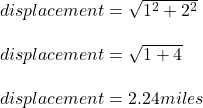## You travel east for 20 miles and then west for 10 miles. What is your average speed and velocity if the entire trip takes 1 hour. Answ

Question

You travel east for 20 miles and then west for 10 miles. What is your average speed and velocity if the entire
trip takes 1 hour. Answer in mile/hour.

in progress 0
6 months 2021-08-03T01:10:17+00:00 1 Answers 5 views 0

## 30 miles/hour, and 2.24 miles/hour

Explanation:

What is average speed?

It is the total distance traveled divided by the time taken to cover the total distance traveled

Given that the various distances are

20 miles and 10 miles

the total distance traveled is 20+10= 30 miles

the average speed is expressed as

average speed= total distance/time taken

average speed= 30/1

average speed= 30 miles/hour

The average velocity is a vector quantity

the velocity is the average displacement divided by time takenaverage velocity= displacement / time

average velocity= 2.24 /1

average velocity= 2.24 miles/hour